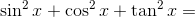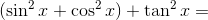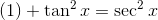# High School Math : Using Pythagorean Identities

## Example Questions

### Example Question #5 : Trigonometric Identities

Simplify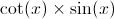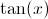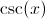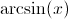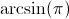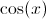Explanation: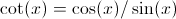.  Thus: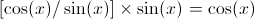### Example Question #6 : Trigonometric Identities

Simplify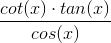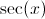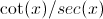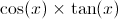Explanation: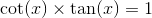and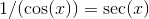.

### Example Question #7 : Trigonometric Identities

Simplify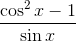.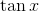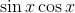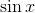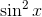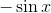Explanation:

Remember that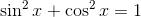. We can rearrange this to simplify our given equation: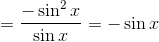### Example Question #8 : Trigonometric Identities

Simplify: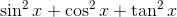This is the most simplified version.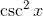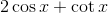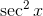Explanation:

Whenever you see a trigonometric function squared, start looking for a Pythagorean identity.

The two identities used in this problem areand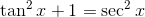.

Substitute and solve.